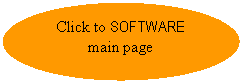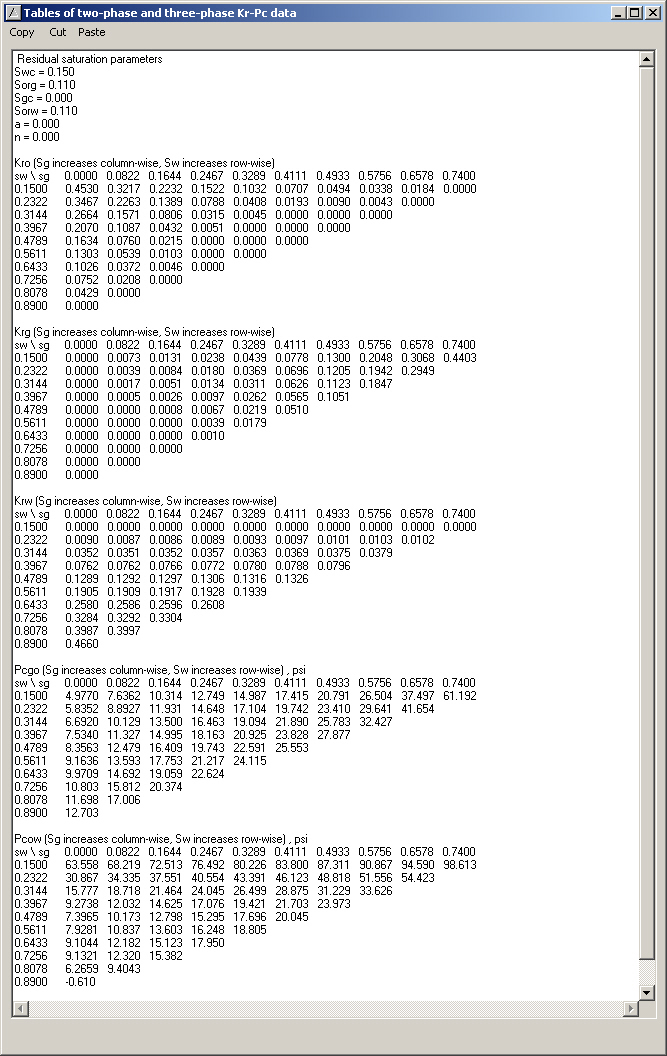The MSF-Maker software featured in this www.msfmaker08.com website has involved the programming/codes (amongst other things) of the following (or similar) displays. If you are interested to receive programming codes (in Visual Basic), or just need some programming assistance, please email us at msfmaker@gmail.com:

1. Ternary diagrams with phase saturations at 6 different positions (Figure 1).

1. X-Y plots (normal, semi-logs and log-log plots), Figure 2.

1. Two-phase relative permeability and capillary pressure plots (Figure 3). These require correct normalization and de-normalization techniques/equations.

1. Displays of Kr and PC data with inputs from a database (Figure 4).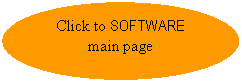1. Two-dimensional tabular data (Figure 5).

Figure 1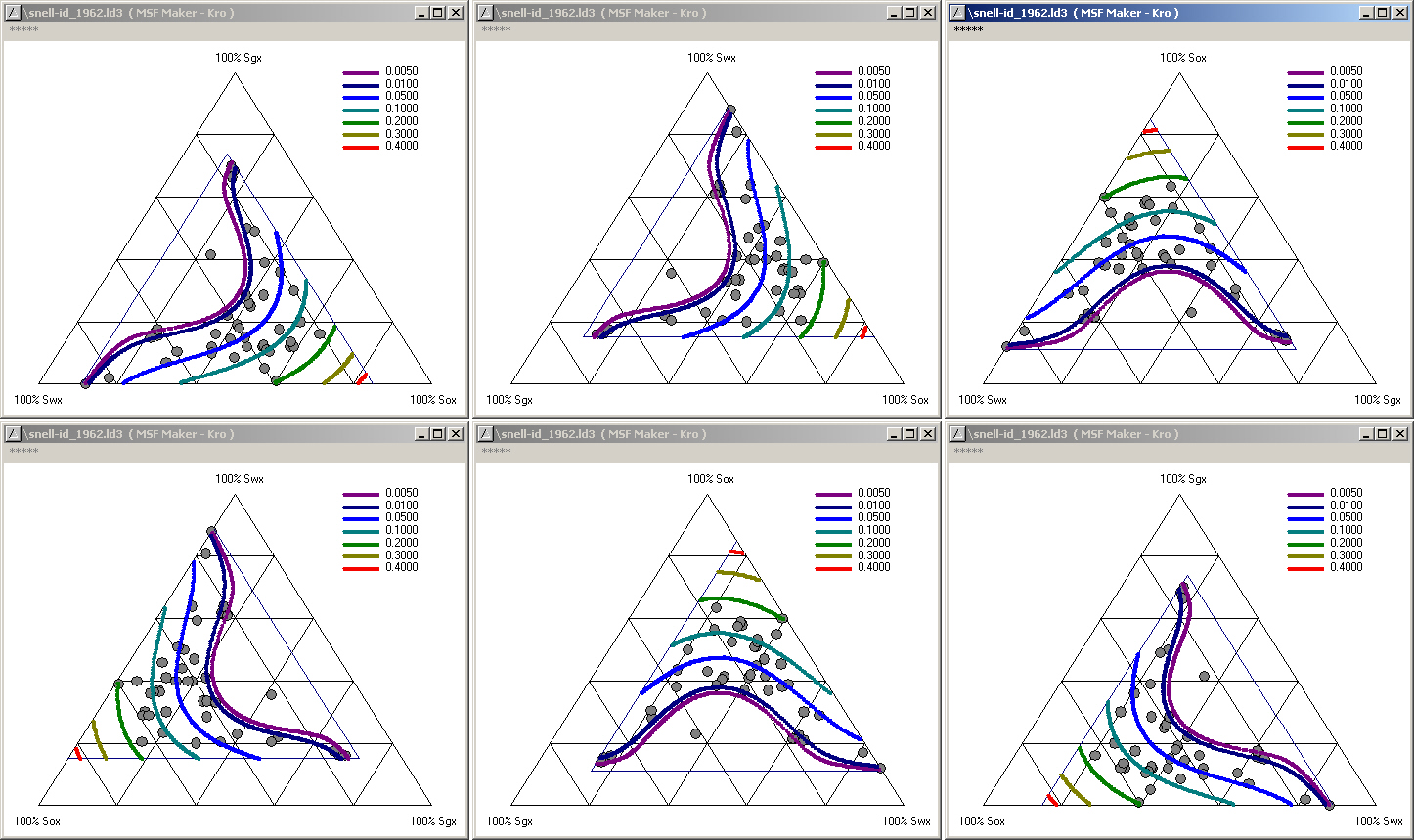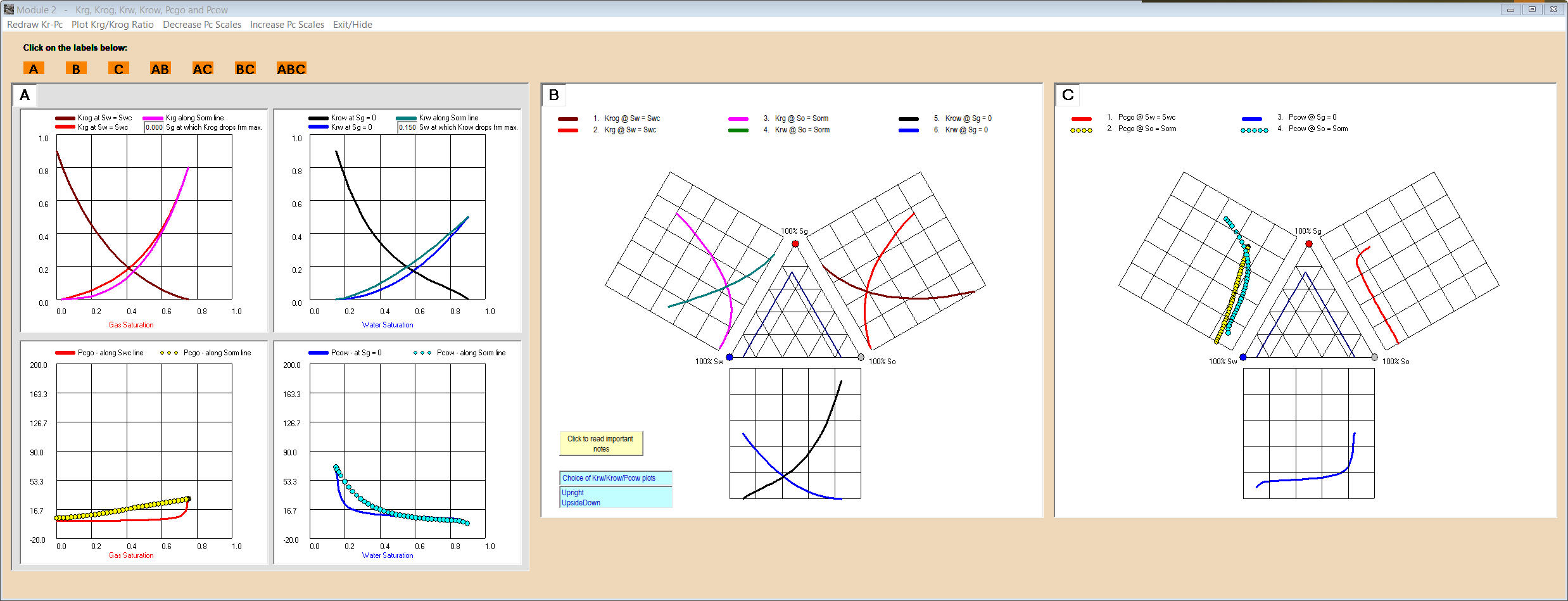Figure 2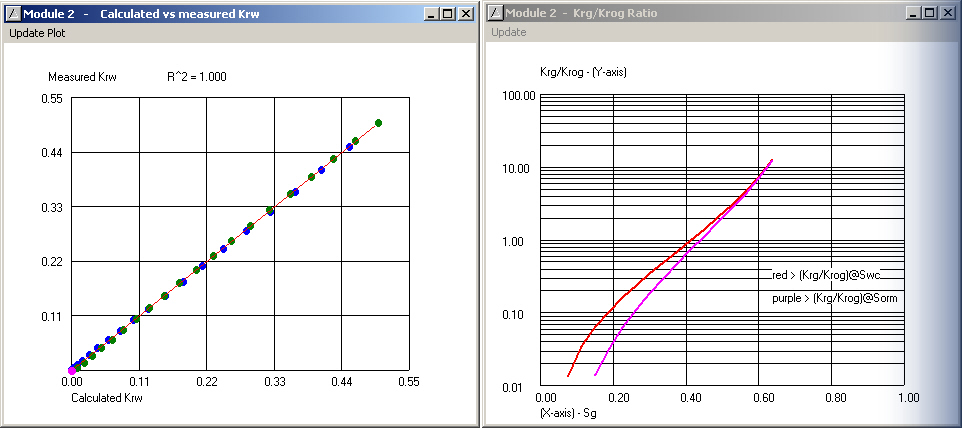3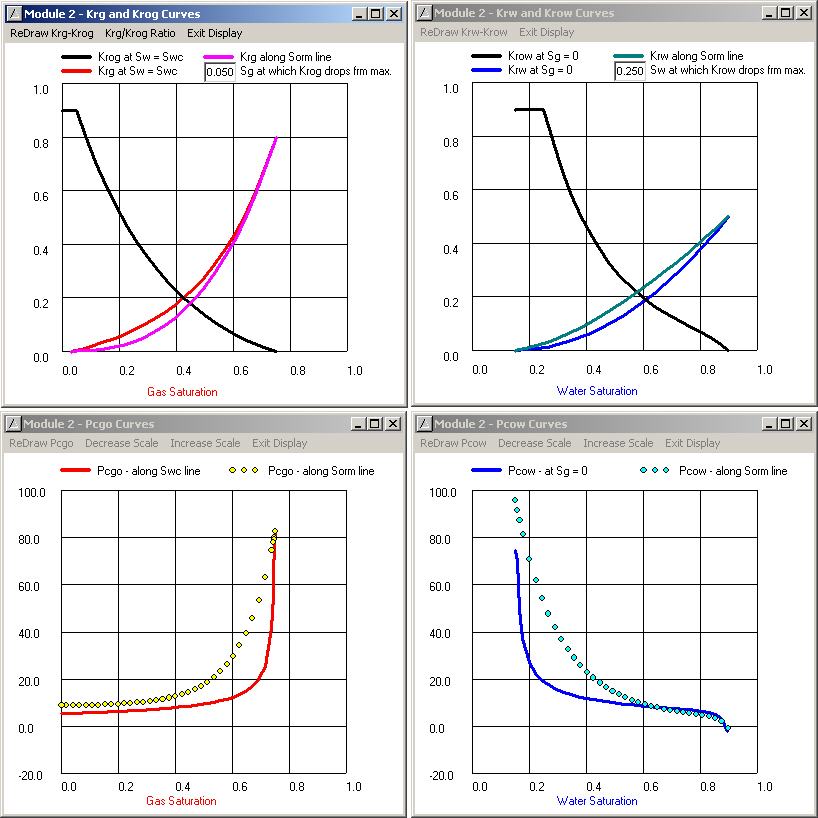45